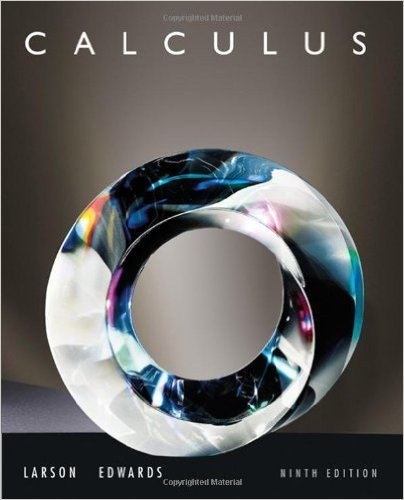×
×

# Solutions for Chapter P2: Linear Models and Rates of Change## Full solutions for Calculus | 9th Edition

ISBN: 9780547167022Solutions for Chapter P2: Linear Models and Rates of Change

Solutions for Chapter P2
4 5 0 351 Reviews
18
3
##### ISBN: 9780547167022

Calculus was written by and is associated to the ISBN: 9780547167022. This expansive textbook survival guide covers the following chapters and their solutions. Chapter P2: Linear Models and Rates of Change includes 100 full step-by-step solutions. Since 100 problems in chapter P2: Linear Models and Rates of Change have been answered, more than 67374 students have viewed full step-by-step solutions from this chapter. This textbook survival guide was created for the textbook: Calculus , edition: 9.

Key Calculus Terms and definitions covered in this textbook
• Arc length formula

The length of an arc in a circle of radius r intercepted by a central angle of u radians is s = r u.

• Arctangent function

See Inverse tangent function.

• Base

See Exponential function, Logarithmic function, nth power of a.

• Compounded continuously

Interest compounded using the formula A = Pert

• Descriptive statistics

The gathering and processing of numerical information

• Endpoint of an interval

A real number that represents one “end” of an interval.

• First-degree equation in x , y, and z

An equation that can be written in the form.

• Heron’s formula

The area of ¢ABC with semiperimeter s is given by 2s1s - a21s - b21s - c2.

• Inferential statistics

Using the science of statistics to make inferences about the parameters in a population from a sample.

• Integrable over [a, b] Lba

ƒ1x2 dx exists.

• Inverse properties

a + 1-a2 = 0, a # 1a

• Lemniscate

A graph of a polar equation of the form r2 = a2 sin 2u or r 2 = a2 cos 2u.

• Mapping

A function viewed as a mapping of the elements of the domain onto the elements of the range

• Ordered set

A set is ordered if it is possible to compare any two elements and say that one element is “less than” or “greater than” the other.

• Parabola

The graph of a quadratic function, or the set of points in a plane that are equidistant from a fixed point (the focus) and a fixed line (the directrix).

• Polar coordinate system

A coordinate system whose ordered pair is based on the directed distance from a central point (the pole) and the angle measured from a ray from the pole (the polar axis)

• Reflexive property of equality

a = a

• Solve by substitution

Method for solving systems of linear equations.

• Sum of complex numbers

(a + bi) + (c + di) = (a + c) + (b + d)i

• Wrapping function

The function that associates points on the unit circle with points on the real number line

×